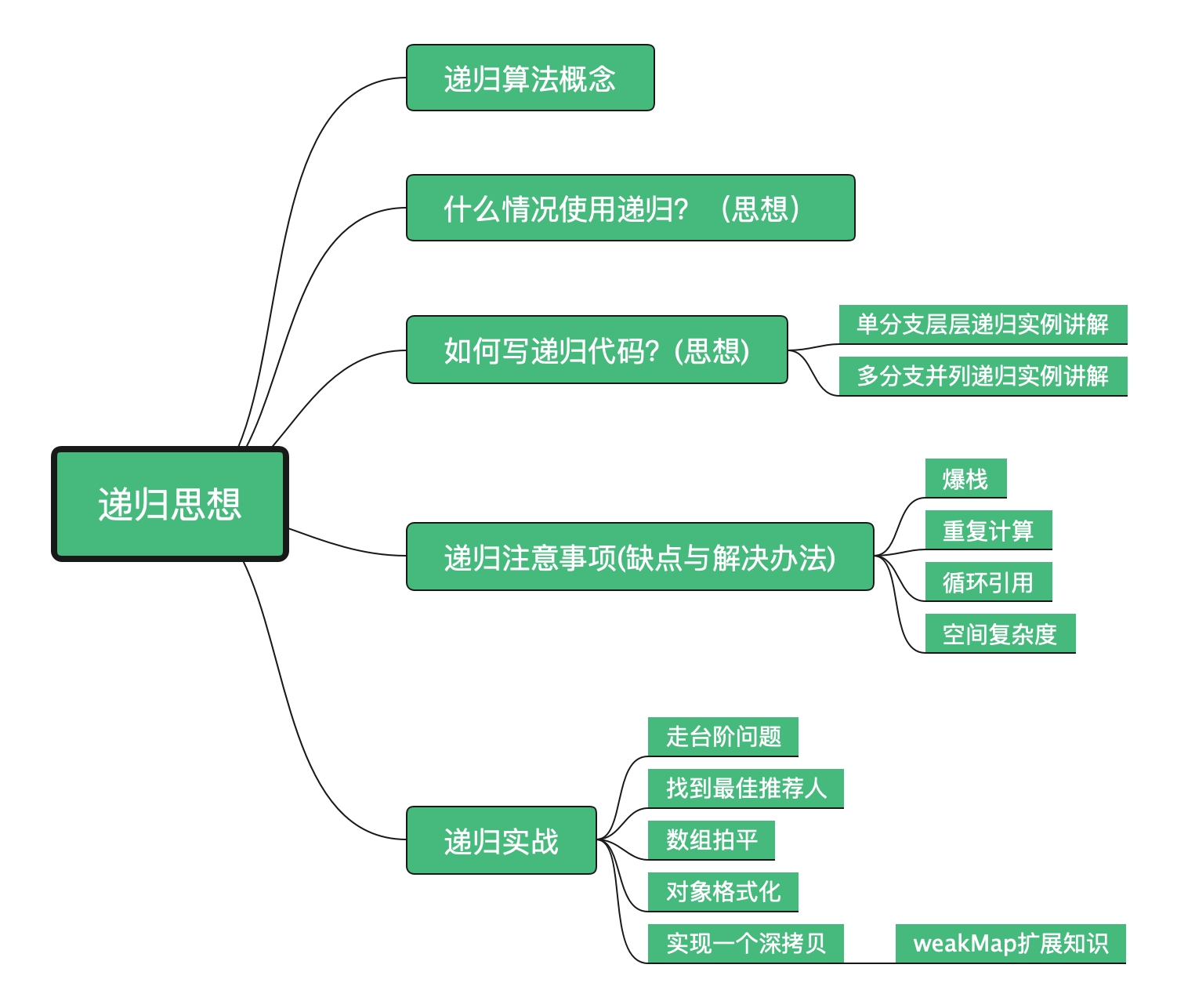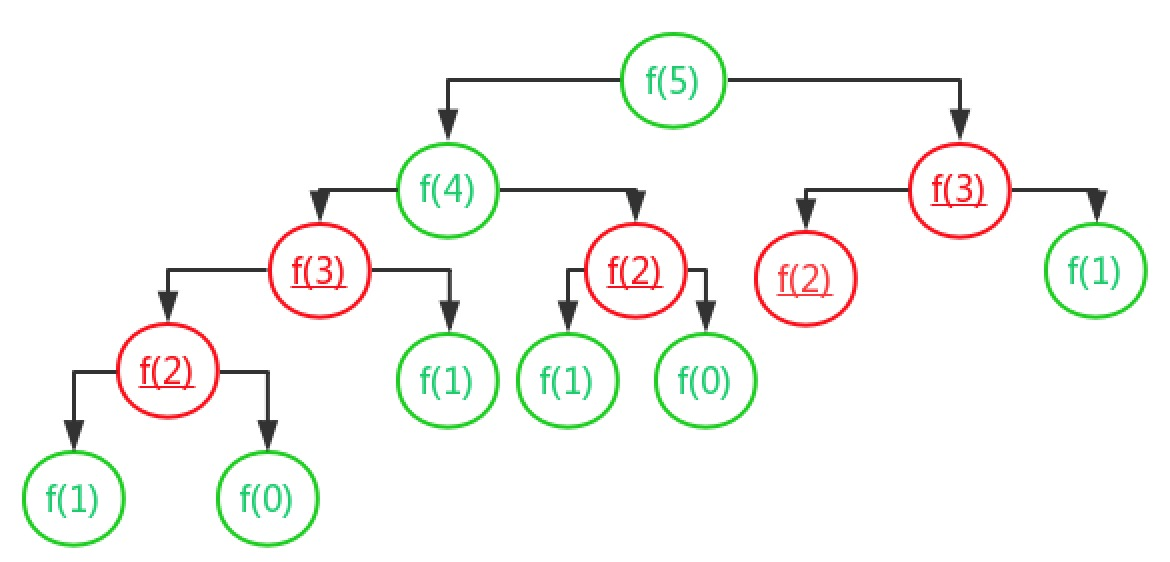# 聊聊面试必考-递归思想与实战2019-10-9Javascript

## # 本篇文章你将学到## # 为什么要写这篇文章

1. “递归”算法对于一个程序员应该算是最经典的算法之一，而且它越想越乱，很多复杂算法的实现也都用到了递归，例如深度优先搜索，二叉树遍历等。
2. 面试中常常会问递归相关的内容(深拷贝，对象格式化，数组拍平，走台阶问题等)
3. 最近项目中有一个需求，裂变分享，但是不仅仅给分享人返利，还会给最终分享人返利，但是只做到 4 级分销（也用到了递归，文中会讲解）

## # 递归算法是什么

`维基百科`: `递归`是在一个函数定义的内部用到自身。有此种定义的函数叫做递归。听起来好像会导致无限重复，但只要定义适当，就不会这样。 一般来说，一个递归函数的定义有两个部分。首先，至少要有一个底线，就是一个简单的线，越过此处，`递归`## # 递归算法思想讲解用和注意事项

### # 什么时候使用递归?

#### # 满足递归的条件

1. 一个问题的解可以分解为几个子问题的解。

1. 这个问题分解之后的子问题，除了数据规模不同，求解思路完全一样

1. 存在递归终止条件

### # 如何写递归代码？(满足上面条件，确认使用递归后)

1. 写出递归公式(注意几分支递归)

2. 找到终止条件

#### # 分析排队取票的例子(`单分支层层递归`)

``````f(n) = f(n - 1) + 1
//f(n) 为我所在的当前层
//f(n-1) 为我前面的人所在的当前层
// +1 为我前面层与我所在层
``````
1
2
3
4

#### # 再看一个走台阶例子(`多分支并列递归`)

：假设有 n 个台阶，每次你可以跨一个台阶或者两个台阶，请问走这 n 个台阶有多少种走法？用编程求解。

``````f(n) = f(n - 1) + f(n - 2)
``````
1

`n=2` 时，`f(2)=f(1)+f(0)`。如果递归终止条件只有一个 `f(1)=1`，那 `f(2)` 就无法求解了。所以除了 `f(1)=1` 这一个递归终止条件外，还要有 `f(0)=1`，表示走 0 个台阶有一种走法，不过这样子看起来就不符合正常的逻辑思维了。所以，我们可以把 `f(2)=2` 作为一种终止条件，表示走 2 个台阶，有两种走法，一步走完或者分两步来走。

``````f(1) = 1
f(2) = 2
f(n) = f(n - 1) + f(n - 2)
``````
1
2
3

``````function walk(n) {
if (n === 1) return 1
if (n === 2) return 2
return f(n - 1) + f(n - 2)
}
``````
1
2
3
4
5

### #写递归代码时注意事项

#### # 1. 爆栈

``````function f(n) {
if (n === 1) return 1
return f(n - 1) + 1
}
``````
1
2
3
4

``````// 全局变量，表示递归的深度。
let depth = 0;

function f(n) {
++depth；
if (depth > 1000) throw exception;

if (n == 1) return 1;
return f(n-1) + 1;
}
``````
1
2
3
4
5
6
7
8
9
10

#### # 2.重复计算

``````function walk(n) {
if (n === 1) return 1
if (n === 2) return 2
return walk(n - 1) + walk(n - 2)
}
``````
1
2
3
4
5``````let mapData = new Map()
function walk(n) {
if (n === 1) return 1
if (n === 2) return 2
// 值的判断和存储
if (mapData.get(n)) {
return setDatas.get(n)
}
let value = walk(n - 1) + walk(n - 2)
mapData.set(n, value)
return value
}
``````
1
2
3
4
5
6
7
8
9
10
11
12

#### # 3.循环引用

``````const target = {
field1: 1,
field2: undefined,
field3: {
child: 'child',
},
field4: [2, 4, 8],
}
target.target = target
``````
1
2
3
4
5
6
7
8
9

### # 递归算法的一点感悟

• 递归算法优点：代码的表达力很强，写起来很简洁。
• 递归算法缺点：递归算法有堆栈溢出(爆栈)的风险、存在重复计算，过多的函数调用会耗时较多等问题(写递归算法的时候一定要考虑这几个缺点)、递归时函数的变量的存储需要额外的栈空间，当递归深度很深时，需要额外的内存占空间就会很多，所以递归有非常高的空间复杂度。

## # 递归算法使用场景(开篇提到的几个面试题)

### # 2.四级分销-找到最佳推荐人

``````let deep = 0;
function findRootReferrerId(actorId) {
deep++;
let referrerId = select referrer_id from [table] where actor_id = actorId;
if (deep === 4) return actorId; // 终止条件
return findRootReferrerId(referrerId);
}
``````
1
2
3
4
5
6
7

1. 数据库中没有`脏数据`(脏数据可能是测试直接手动插入数据产生的，比如 A 推荐了 B，B 又推荐了 A，造成死循环，循环引用)。
2. 确认推荐人插入表中数据的时候，一定判断二者之前的推荐关系是否已经存在。

### # 3.数组拍平

``````let a = [1, 2, 3, [1, 2, [1.4], [1, 2, 3]]]
``````
1

``````function flat(a = [], result = []) {
a.forEach((item) => {
console.log(Object.prototype.toString.call(item))
if (Object.prototype.toString.call(item) === '[object Array]') {
result = result.concat(flat(item, []))
} else {
result.push(item)
}
})
return result
}
console.log(flat(a)) // 输出结果 [ 1, 2, 3, 1, 2, 1.4, 1, 2, 3 ]
``````
1
2
3
4
5
6
7
8
9
10
11
12

### # 4.对象格式化

``````// 格式化对象 大写变为小写
let obj = {
a: '1',
b: {
c: '2',
D: {
E: '3',
},
},
}
function keysLower(obj) {
let reg = new RegExp('([A-Z]+)', 'g')
for (let key in obj) {
if (Object.prototype.hasOwnProperty.call(obj, key)) {
let temp = obj[key]
if (reg.test(key.toString())) {
temp = obj[
key.replace(reg, function(result) {
return result.toLowerCase()
})
] = obj[key]
delete obj[key]
}
if (Object.prototype.toString.call(temp) === '[object Object]') {
keysLower(temp)
}
}
}
return obj
}
console.log(keysLower(obj)) //输出结果 { a: '1', b: { c: '2', d: { e: '3' } } }
``````
1
2
3
4
5
6
7
8
9
10
11
12
13
14
15
16
17
18
19
20
21
22
23
24
25
26
27
28
29
30
31

### # 5.实现一个深拷贝

``````const target = {
field1: 1,
field2: undefined,
field3: {
child: 'child',
},
field4: [2, 4, 8],
}
target.target = target
``````
1
2
3
4
5
6
7
8
9

``````function clone(target, map = new WeakMap()) {
if (typeof target === 'object') {
let cloneTarget = Array.isArray(target) ? [] : {}
if (map.get(target)) {
return map.get(target)
}
map.set(target, cloneTarget)
for (const key in target) {
cloneTarget[key] = clone(target[key], map)
}
return cloneTarget
} else {
return target
}
}
``````
1
2
3
4
5
6
7
8
9
10
11
12
13
14
15

• 检查 map 中有无克隆过的对象
• 有,直接返回
• 没有, 将当前对象作为 key，克隆对象作为 value 进行存储
• 继续克隆

#### # weakMap 补充知识

WeakMap 对象虽然也是一组键/值对的集合，其中的键是弱引用的。其键必须是对象，而值可以是任意的。

## # 参考文章

• 天明夜尽:https://juejin.im/post/5d1dab346fb9a07ec63b3249
• 极客时间的递归学习# # 给我留言

#### 关注作者公众#### 加入技术交流群